Custom SearchFrequency Modulation In frequency modulation, the instantaneous frequency of the radio-frequency wave is varied in accordance with the modulating signal, as shown in view (A) of figure 2-5. As mentioned earlier, the amplitude is kept constant. This results in oscillations similar to those illustrated in view (B). The number of times per second that the instantaneous frequency is varied from the average (carrier frequency) is controlled by the frequency of the modulating signal. The amount by which the frequency departs from the average is controlled by the amplitude of the modulating signal. This variation is referred to as the FREQUENCY DEVIATION of the frequency-modulated wave. We can now establish two clear-cut rules for frequency deviation rate and amplitude in frequency modulation: Figure 2-5. - Effect of frequency modulation on an rf carrier.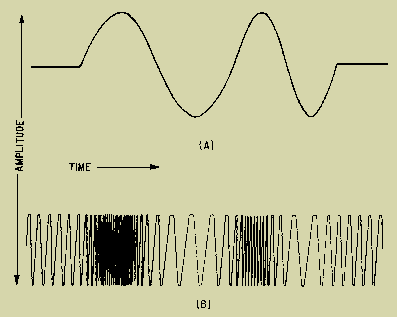AMOUNT OF FREQUENCY SHIFT IS PROPORTIONAL TO THE AMPLITUDE OF THE MODULATING SIGNAL (This rule simply means that if a 10-volt signal causes a frequency shift of 20 kilohertz, then a 20-volt signal will cause a frequency shift of 40 kilohertz.) RATE OF FREQUENCY SHIFT IS PROPORTIONAL TO THE FREQUENCY OF THE MODULATING SIGNAL (This second rule means that if the carrier is modulated with a 1-kilohertz tone, then the carrier is changing frequency 1,000 times each second.) Figure 2-6 illustrates a simple oscillator circuit with the addition of a condenser microphone (M) in shunt with the oscillator tank circuit. Although the condenser microphone capacitance is actually very low, the capacitance of this microphone will be considered near that of the tuning capacitor (C). The frequency of oscillation in this circuit is, of course, determined by the LC product of all elements of the circuit; but, the product of the inductance (L) and the combined capacitance of C and M are the primary frequency components. When no sound waves strike M, the frequency is the rf carrier frequency. Any excitation of M will alter its capacitance and, therefore, the frequency of the oscillator circuit. Figure 2-7 illustrates what happens to the capacitance of the microphone during excitation. In view (A), the audio-frequency wave has three levels of intensity, shown as X, a whisper; Y, a normal voice; and Z, a loud voice. In view (B), the same conditions of intensity are repeated, but this time at a frequency twice that of view (A). Note in each case that the capacitance changes both positively and negatively; thus the frequency of oscillation alternates both above and below the resting frequency. The amount of change is determined by the change in capacitance of the microphone. The change is caused by the amplitude of the sound wave exciting the microphone. The rate at which the change in frequency occurs is determined by the rate at which the capacitance of the microphone changes. This rate of change is caused by the frequency of the sound wave. For example, suppose a 1,000-hertz tone of a certain loudness strikes the microphone. The frequency of the carrier will then shift by a certain amount, say plus and minus 40 kilohertz. The carrier will be shifted 1,000 times per second. Now assume that with its loudness unchanged, the frequency of the tone is changed to 4,000 hertz. The carrier frequency will still shift plus and minus 40 kilohertz; but now it will shift at a rate of 4,000 times per second. Likewise, assume that at the same loudness, the tone is reduced to 200 hertz. The carrier will continue to shift plus and minus 40 kilohertz, but now at a rate of 200 times per second. If the loudness of any of these modulating tones is reduced by one-half, the frequency of the carrier will be shifted plus and minus 20 kilohertz. The carrier will then shift at the same rate as before. This fulfills all requirements for frequency modulation. Both the frequency and the amplitude of the modulating signal are translated into variations in the frequency of the rf carrier. Figure 2-6. - Oscillator circuit illustrating frequency modulation.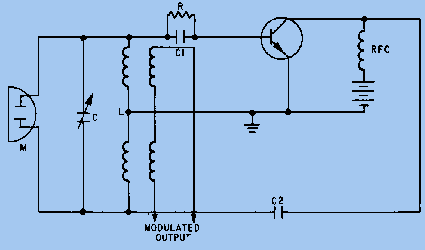Figure 2-7A. - Capacitance change in an oscillator circuit during modulation. CHANGE IN INTENSITY OF SOUND WAVES CHANGES CAPACITY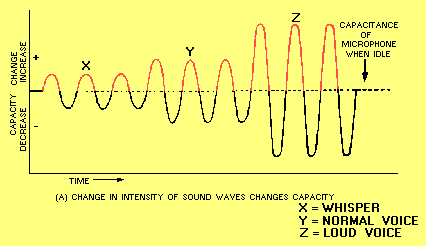Figure 2-7B. - Capacitance change in an oscillator circuit during modulation. AT A FREQUENCY TWICE THAT OF (A), THE CAPACITY CHANGES THE SAME AMOUNT, BUT TWICE AS OFTEN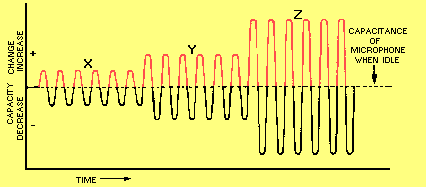Figure 2-8 shows how the frequency shift of an fm signal goes through the same variations as does the modulating signal. In this figure the dimension of the constant amplitude is omitted. (As these remaining waveforms are presented, be sure you take plenty of time to study and digest what the figures tell you. Look each one over carefully, noting everything you can about them. Doing this will help you understand this material.) If the maximum frequency deviation is set at 75 kilohertz above and below the carrier, the audio amplitude of the modulating wave must be so adjusted that its peaks drive the frequency only between these limits. This can then be referred to as 100-PERCENT MODULATION, although the term is only remotely applicable to fm. Projections along the vertical axis represent deviations in frequency from the resting frequency (carrier) in terms of audio amplitude. Projections along the horizontal axis represent time. The distance between A and B represents 0.001 second. This means that carrier deviations from the resting frequency to plus 75 kilohertz, then to minus 75 kilohertz, and finally back to rest would occur 1,000 times per second. This would equate to an audio frequency of 1,000 hertz. Since the carrier deviation for this period (A to B) extends to the full allowable limits of plus and minus 75 kilohertz, the wave is fully modulated. The distance from C to D is the same as that from A to B, so the time interval and frequency are the same as before. Notice, however, that the amplitude of the modulating wave has been decreased so that the carrier is driven to only plus and minus 37.5 kilohertz, one-half the allowable deviation. This would correspond to only 50-percent modulation if the system were AM instead of fm. Between E and F, the interval is reduced to 0.0005 second. This indicates an increase in frequency of the modulating signal to 2,000 hertz. The amplitude has returned to its maximum allowable value, as indicated by the deviation of the carrier to plus and minus 75 kilohertz. Interval G to H represents the same frequency at a lower modulation amplitude (66 percent). Notice the GUARD BANDS between plus and minus 75 kilohertz and plus and minus 100 kilohertz. These bands isolate the modulation extremes of this particular channel from that of adjacent channels. Figure 2-8. - Frequency-modulating signal.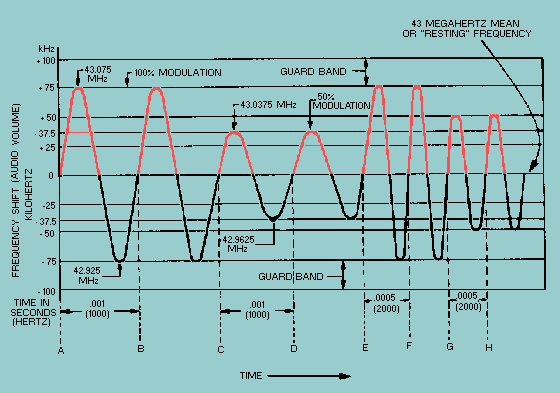Integrated Publishing, Inc. - A (SDVOSB) Service Disabled Veteran Owned Small Business